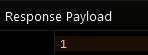# Get Object Count¶

The Object Count API provides a way to obtain the following values from the server:

• Number of objects in a table
• Number of objects matching query
• Number of related objects

### Method¶

``````Future<int> Backendless.data.of("TABLE-NAME").getObjectCount([DataQueryBuilder queryBuilder]);
``````
``````Future<int> Backendless.data.withClass<E>().getObjectCount([DataQueryBuilder queryBuilder]);
``````

where:

Argument                Description
`TABLE-NAME` Name of the table where to calculate the object count.
`E` Java class of the data object which identifies the table where to calculate the object count.
`queryBuilder` Instance of com.backendless.persistence.DataQueryBuilder. When present in the arguments, the object must contain a whereClause query. The query is used by the server to identify a collection of objects.

### Return Value¶

The integer value which is the object count.

### Example¶

#### Total object count for a table¶

The following sample request retrieves total number of objects in table `Order`:

``````Backendless.data.of("Order").getObjectCount().then((count) {
print("Total objects in the Order table - \$count");
});
``````
``````import 'package:backendless_sdk/backendless_sdk.dart';

@reflector
class Order {
String objectId;
int orderAmount;
}

Backendless.data.withClass<Order>().getObjectCount().then((count) {
print("Total objects in the Order table - \$count");
});
``````

#### Object count for a query¶

The following sample request retrieves total number of objects in table `Order` which satisfy the condition of `orderAmount > 100`:

``````DataQueryBuilder queryBuilder = DataQueryBuilder()
..whereClause = "orderAmount > 100";

Backendless.data.of("Order").getObjectCount(queryBuilder).then((count) {
print("Found objects - \$count");
});
``````
``````DataQueryBuilder queryBuilder = DataQueryBuilder()
..whereClause = "orderAmount > 100";

Backendless.data.withClass<Order>().getObjectCount(queryBuilder).then((count) {
print("Total objects in the Order table - \$count");
});
``````

The following sample request retrieves total number of related "child" objects for a parent object. The parent table is `Person`. It contains a relation column called `address` pointing to the `Address`table. The query below retrieves a count of related child objects for a parent object with `objectID` of `XXXX-XXXX-XXXX-XXXX`. The whereClause query syntax for this scenario is:

`Person[address].objectId = 'XXXX-XXXX-XXXX-XXXX'`

``````DataQueryBuilder queryBuilder = DataQueryBuilder()

print("Found objects - \$count");
});
``````
``````DataQueryBuilder queryBuilder = DataQueryBuilder()

print("Found objects - \$count");
});
``````

### Codeless Reference¶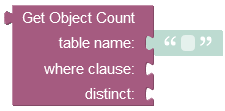where:

Argument                Description
`table name` Name of the data table where to calculate the object count.
`where clause` Optional argument. If set, it is a search query used by the server it to determine the number of objects matching the condition. Refer to the Search With The Where Clause topic for more information.
`distinct` Used to return unique objects from the data table. Only custom properties/columns are considered in the query. Hence, if the data table contains a duplicate object, then it is not counted in the operation.

Returns the number of objects in a table (if the `where clause` is not set), otherwise the number of objects matching the search query.

Consider the following records in the `employees` data table: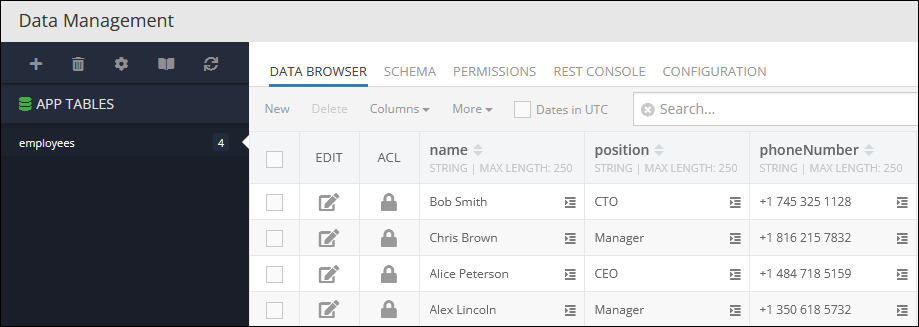The example below counts specific objects in the data table since the `where clause` condition is set to exclude objects that contain the value `'Manager'` in the column `position`. Thereby, objects with the `'Manager'` values in the `position` column are not counted in the operation.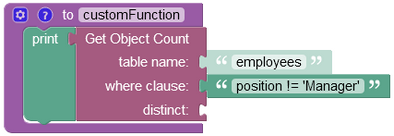The result of this operation will look as shown below after the Codeless logic runs:Consider the data table below that contains two duplicate objects: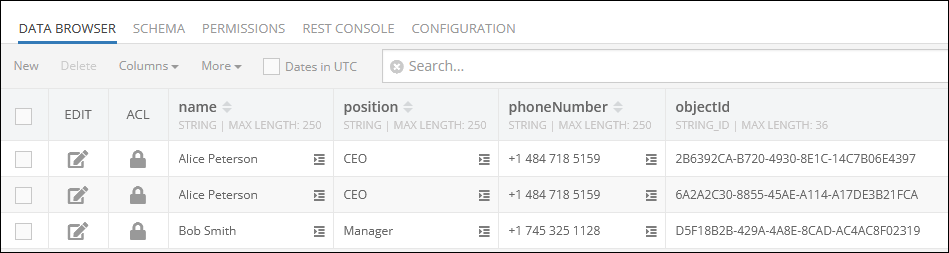The Codeless logic below has a `where clause` condition which is set to include in the operation only those objects that contain the value `'CEO'` in the `position` column. The `distinct` parameter is set to `true`, hence the operation is set to count only unique objects, duplicates are skipped.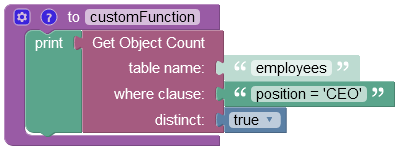The result of this operation will look as shown below after the Codeless logic runs: